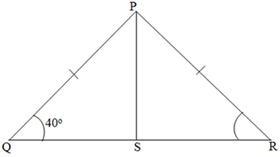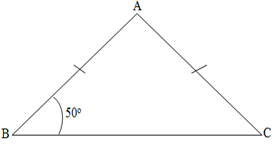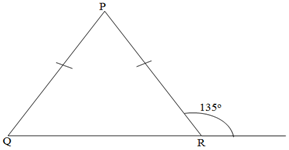# MCQ Questions for Class 9 Maths Chapter 7 Triangles with Answers

## MCQ Questions for Class 9 Maths Chapter 7 Triangles with Answers

MCQs from Class 9 Maths Chapter 7 – Triangles are provided here to help students prepare for their upcoming Maths exam.

MCQs from CBSE Class 9 Maths Chapter 7: Triangles

Q1. If E and F are the midpoints of equal sides AB and AC of a triangle ABC. Then:
a) BF=AC
b) BF=AF
c) CE=AB
d) BF = CE

Explanation: AB and AC are equal sides.

AB = AC (Given)

∠A = ∠A (Common angle)

AE = AF (Halves of equal sides)

∆ ABF ≅ ∆ ACE (By SAS rule)

Hence, BF = CE (CPCT)

Q2. ABC is an isosceles triangle in which altitudes BE and CF are drawn to equal sides AC and AB respectively. Then:
a) BE>CF
b) BE<CF
c) BE=CF
d) None of the above

Explanation:

∠A = ∠A (common arm)

∠AEB = ∠AFC (Right angles)

AB = AC (Given)

∴ ΔAEB ≅ ΔAFC

Hence, BE = CF (by CPCT)

Q3. If ABC and DBC are two isosceles triangles on the same base BC. Then:
a) ∠ABD = ∠ACD
b) ∠ABD > ∠ACD
c) ∠ABD < ∠ACD
d) None of the above

AB = AC (Sides of isosceles triangle)

BD = CD (Sides of isosceles triangle)

So, ΔABD ≅ ΔACD.

∴ ∠ABD = ∠ACD (By CPCT)

Q4. If ABC is an equilateral triangle, then each angle equals to:
a) 90°
B)180°
c) 120°
d) 60°

Explanation: Equilateral triangle has all its sides equal and each angle measures 60°.

AB= BC = AC (All sides are equal)

Hence, ∠A = ∠B = ∠C (Opposite angles of equal sides)

Also, we know that,

∠A + ∠B + ∠C = 180°

⇒ 3∠A = 180°

⇒ ∠A = 60°

∴ ∠A = ∠B = ∠C = 60°

Q5. If AD is an altitude of an isosceles triangle ABC in which AB = AC. Then:
a) BD=CD
b) BD>CD
c) BD<CD
d) None of the above

Explanation: In ΔABD and ΔACD,

AB = AC (Given)

∴ ΔABD ≅ ΔACD (By RHS congruence condition)

BD = CD (By CPCT)

Q6. In a right triangle, the longest side is:
a) Perpendicular
b) Hypotenuse
c) Base
d) None of the above

Explanation: In triangle ABC, right-angled at B.

∠B = 90

By angle sum property, we know:

∠A + ∠B + ∠C = 180

Hence, ∠A + ∠C = 90

So, ∠B is the largest angle.

Therefore, the side (hypotenuse) opposite to largest angle will be longest one.

Q7. In triangle ABC, if AB=BC and ∠B = 70, ∠A will be:
a) 70
b) 110
c) 55
d) 130

Explanation: Given,

AB = BC

Hence, ∠A=∠C

And ∠B = 70

By angle sum property of triangle we know:

∠A+∠B+∠C = 180

2∠A+∠B=180

2∠A = 180-∠B = 180-70 = 110

∠A = 55

Q8. For two triangles, if two angles and the included side of one triangle are equal to two angles and the included side of another triangle. Then the congruency rule is:
a) SSS
b) ASA
c) SAS
d) None of the above

(b)

Q9. A triangle in which two sides are equal is called:
a) Scalene triangle
b) Equilateral triangle
c) Isosceles triangle
d) None of the above

(c)

Q10. The angles opposite to equal sides of a triangle are:
a) Equal
b) Unequal
c) supplementary angles
d) Complementary angles

(a)

Q11. In two triangles DEF and PQR, if DE = QR, EF = PR and FD = PQ, then
a) ∆DEF ≅ ∆PQR
b) ∆FED ≅ ∆PRQ
c) ∆EDF ≅ ∆RPQ
d) ∆PQR ≅ ∆EFD

(b)

Q12. In ∆ABC, BC = AB and ∠B = 80°. Then ∠A is equal to:
a) 80o
b) 40o
c) 50o
d) 100o

(c)

Q13. Two sides of a triangle are of length 5 cm and 1.5 cm. The length of the third side of the triangle cannot be:
a) 3.6 cm
b) 4.1 cm
c) 3.8 cm
d) 6.9 cm

(d)

Q14. In ∆PQR, if ∠R > ∠Q, then
a) QR > PR
b) PQ > PR
c) PQ < PR
d) QR < PR

(b)

Q15. D is a point on the side BC of a ΔABC such that AD bisects ∠BAC. Then
a) BD : DC = AB : AC
b) CD > CA
c) BD > BA
d) BA > BD

(a)

Q16. It is given that Δ ABC ≅ Δ FDE and AB = 5 cm, ∠B = 40° and ∠A = 80°. Then which of the following is true?
a) DF = 5 cm, ∠F = 60°
b) DF = 5 cm, ∠E = 60°
c) DE = 5 cm, ∠E = 60°
d) DE = 5 cm, ∠D = 40°

(b)

Q17. All the medians of a triangle are equal in case of a:
a) Scalene triangle
b) Right angled triangle
c) Equilateral triangle
d) Isosceles triangle

(c)

Q18. In the given figure, PS is the median then ∠QPS?a) 40o
b) 50o
c) 80o
d) 90o

(b)

Q19. In triangle PQR if ∠Q = 90°, then:
a) PQ is the longest side
b) QR is the longest side
c) PR is the longest side
d) None of these

(c)

Q20. In the given figure, AB = AC and ∠B = 50° then; ∠A is:a) 50o
b) 80o
c) 100o
d) 130o

(b)

Q21. In the given figure, if the exterior angle is 135o then ∠P is:a) 45o
b) 60o
c) 80o
d) 90o

(d)

Q22. If in ΔPQR, PQ = PR then:
a) ∠P = ∠R
b) ∠P = ∠Q
c) ∠Q = ∠R
d) None of these

(c)

Q23. In a triangle ABC, ∠B = 35° and ∠C = 60°, then
a) ∠A = 80°
b) ∠A = 85°
c) ∠A = 120°
d) ∠A = 145°

(b)

Q24. In triangles ABC and PQR, AB = AC, ∠C = ∠P and ∠B = ∠Q. The two triangles are:
a) Isosceles but not congruent
b) Isosceles and congruent
c) Congruent but not isosceles
d) Neither congruent nor isosceles

(a)

Q25. In triangles ABC and DEF, AB = FD and ∠A = ∠D. The two triangles will be congruent by SAS axiom if:
a) BC = EF
b) AC = DE
c) AC = EF
d) BC = DE